## RS Aggarwal Class 8 Solutions Chapter 12 Direct and Inverse Proportions Ex 12A

These Solutions are part of RS Aggarwal Solutions Class 8. Here we have given RS Aggarwal Solutions Class 8 Chapter 12 Direct and Inverse Proportions Ex 12A.

Other Exercises

Question 1.
Solution:
(i) $$\\ \frac { x }{ y }$$ = $$\\ \frac { 3 }{ 9 }$$ = $$\\ \frac { 1 }{ 3 }$$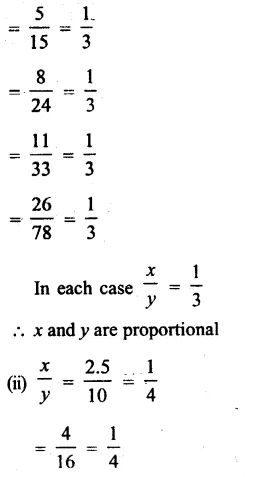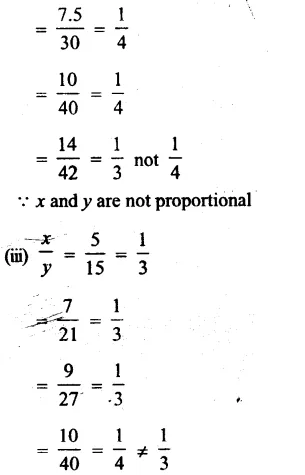Question 2.
Solution:
x and y are directly proportional
$$\\ \frac { x }{ y }$$ = $$\\ \frac { 3 }{ 72 }$$ = $$\\ \frac { 1 }{ 24 }$$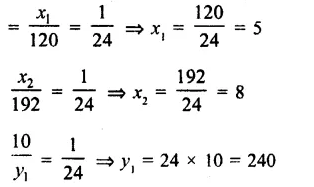Question 3.
Solution:Question 4.
Solution: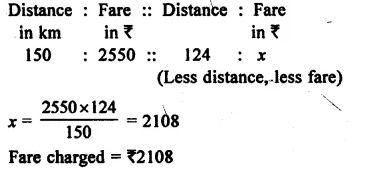Question 5.
Solution:
Let distance covered = x thenQuestion 6.
Solution:
Let no. of dolls = x, then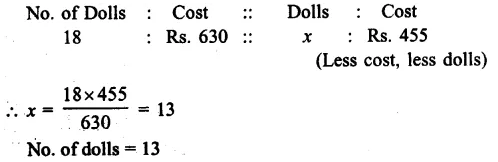Question 7.
Solution:
Let x kg of sugar will be bought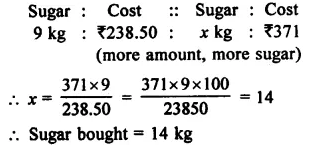Question 8.
Solution:
Let cloth bought = x m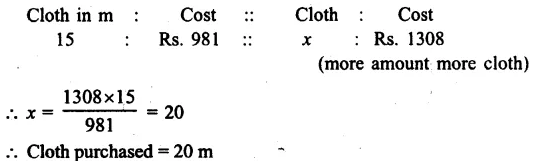Question 9.
Solution:
Let length of model ship = x mQuestion 10.
Solution:
Let x kg dust will be picked up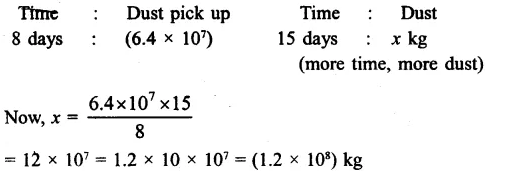Question 11.
Solution:
A speed of car = 50 km/hr
Distance travelled in 1 hr. = 5 m
Let required distance travelled in 1 hr. 12 min.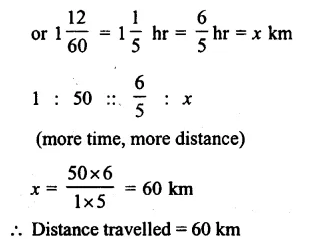Question 12.
Solution:
Let required distance covered = x km
Speed of man = 5 km/hrQuestion 13.
Solution:
Let required thickness = x mmQuestion 14.
Solution:
Let men required = x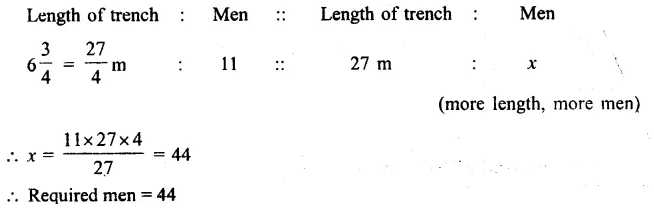Question 15.
Solution:
Let no. of words type in 8 minutes = x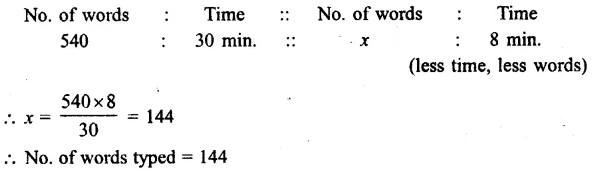Hope given RS Aggarwal Solutions Class 8 Chapter 12 Direct and Inverse Proportions Ex 12A are helpful to complete your math homework.

If you have any doubts, please comment below. Learn Insta try to provide online math tutoring for you.